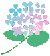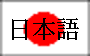#\$BG_1+(B(\$B\$D\$f(B) - 6\$B7n\$+\$i(B7\$B7n(B\$BF|K\\$G\$O!"(B6\$B7n\$+\$i(B7\$B7n\$NCf=\\$^\$G\$N4V!"G_1+(B(\$B\$D\$f(B)\$B\$KF~\$j\$^\$9!#(B6\$B7n\$K\$O=KF|(B \$B\$b9T;v\$b\$J\$/!"\$?\$@G_1+\$@\$1\$J\$N\$G!"B?\$/\$NF|K\?M\$O(B6\$B7n\$r7y\$\$!"\$?\$@\$?\$@B`(B \$B6~4i\$G2a\$4\$7\$^\$9!#(B\$B\$3\$N5(@a\$K4X\$9\$k\$H\$F\$bM-L>\$G4JC1\$GLLGr\$\$(B "\$B\$+\$?\$D\$`\$j(B" \$B\$H!"(B"\$B\$"\$a(B \$B\$U\$j(B"\$B\$H\$\$\$&2N\$,\$"\$j\$^\$9!#\$<\$RJ9\$\$\$FN}=,\$7\$F\$_\$F2<\$5\$\$!#(B